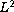Electron. J. Diff. Eqns., Vol. 2007(2007), No. 92, pp. 1-9.

### Continuous dependence for the Brinkman equations of flow in double-diffusive convection Hongliang Tu, Changhao Lin

Abstract:
This paper concerns the structural stability for convective motion in a fluid-saturated porous medium under the Brinkman scheme. Continuous dependence for the solutions on the gravity coefficients and the Soret coefficient are proved. First of all, an a priori bound innorm is derived whereby we show the solution depends continuously in L^2 norm on changes in the gravity coefficients and the Soret coefficient. This estimate also implies that the solutions decay exponentially.

Submitted April 9, 2007. Published June 16, 2007.
Math Subject Classifications: 35B30, 35K55, 35Q35.
Key Words: Continuous dependence; structural stability; gravity coefficients; Soret coefficient; Brinkman equations

Show me the PDF file (196K), TEX file, and other files for this article.Hongliang Tu Department of Applied Mathematics Zhuhai college of Jilin University, 519041, China email: tuhongliang00@126.com Changhao Lin School of Mathematical Sciences South China Normal University, 510631, China email: linchh@scnu.edu.cn